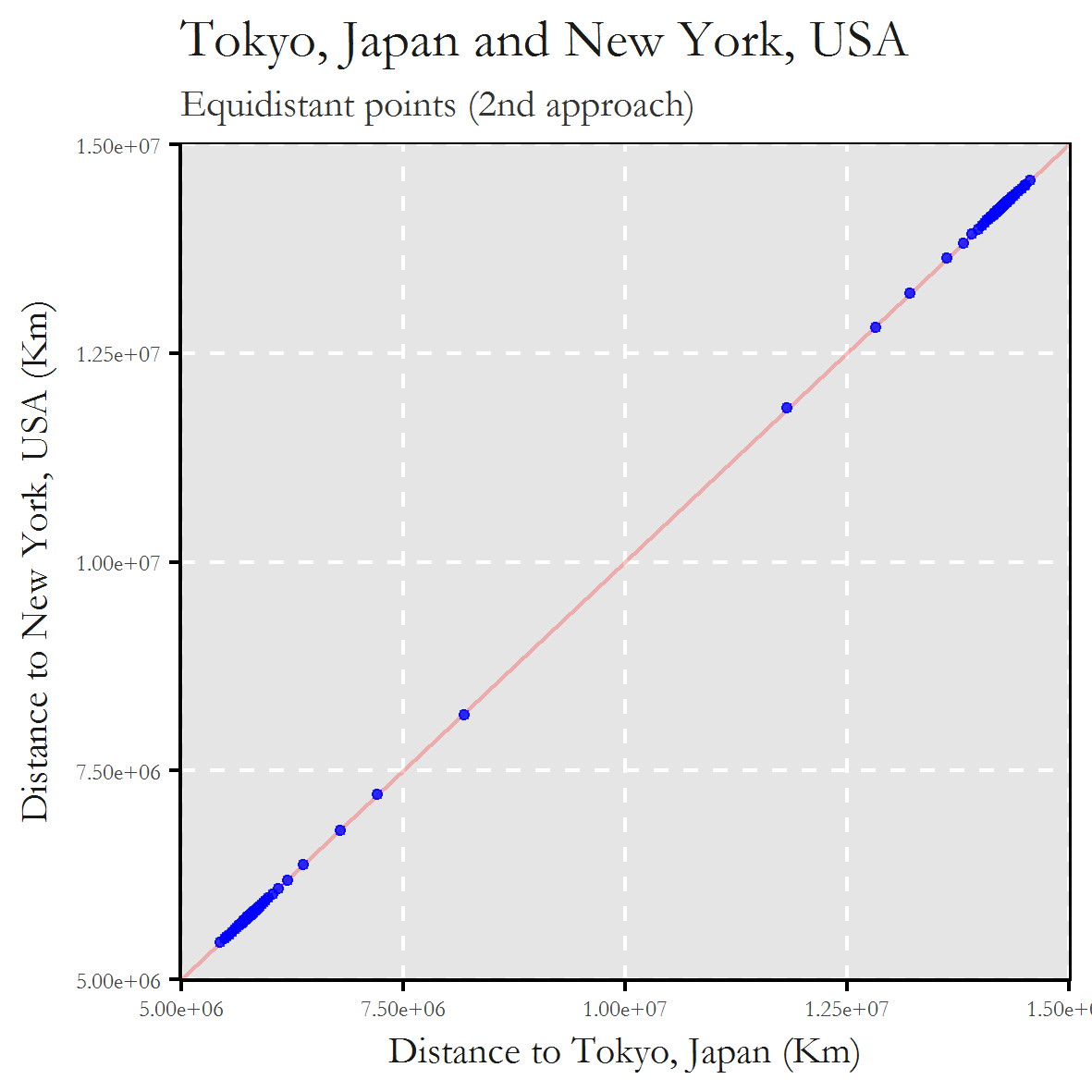# How to Find Equidistant Coordinates Between Two Locations on Earth

February 13, 2017
By

Want to share your content on R-bloggers? click here if you have a blog, or here if you don't.

Here’s to the ones who dream
foolish, as they may seem
(The Fools Who Dream, ‘La La Land’ OST)

One of the key points of The Meeting Point Locator is to obtain an orthogonal great circle to the bearing defined by any two given locations on Earth. A great circle is the intersection of the sphere and a plane that passes through the center point of the sphere. In other words, a great circle is a false meridian. The orthogonal great circle to the direction defined by any two given locations is the one which passes by all equidistant points to both of them (at least this is what I call orthogonal great circle). This was my first approach to obtain it:

• Get the midpoint between the initial locations, let’s call it p1
• Calculate the direction (bearing angle) between the initial locations, let’s call it α
• Obtain a very close point to p1 (only 1 meter away) with bearing α+90, let’s call it p2
• Calculate the great circle which passes through p1 and p2

This is the code I used in this first approach:

```library(dplyr)
library(ggmap)
library(geosphere)
library(leaflet)
library(ggplot2)
library(scales)
library(extrafont)
windowsFonts(Garamond=windowsFont("Garamond"))

#Starting places
place2="Toledo, Spain"

# Call to Google Maps API to obtain coordinates of Starting places
p1=geocode(place1, output = "latlon")
p2=geocode(place2, output = "latlon")

#Midpoint of p1 and p2
mid=midPoint(p1, p2)

#Direction between p1 and p2
bea=bearingRhumb(p1, p2)

# Great circle between midpoint and 1-meter separated point with bearing bea+90
points=greatCircle(destPoint(p=mid, b=bea+90, d=1), mid, n=100)

# Arrange the points dependning on the distance to the input locations
data.frame(dist2p1=apply(points, 1, function (x) distGeo(p1, x)),
dist2p2=apply(points, 1, function (x) distGeo(p2, x))) %>%
cbind(points) -> points

opts=theme(
panel.background = element_rect(fill="gray90"),
panel.border = element_rect(colour="black", fill=NA),
axis.line = element_line(size = 0.5, colour = "black"),
axis.ticks = element_line(colour="black"),
panel.grid.major = element_line(colour="white", linetype = 2),
panel.grid.minor = element_blank(),
axis.text = element_text(colour="gray25", size=6, family = "Garamond"),
axis.title = element_text(size=10, colour="gray10", family = "Garamond"),
legend.key = element_blank(),
legend.position = "none",
legend.background = element_blank(),
plot.title = element_text(size = 14, colour="gray10", family = "Garamond"),
plot.subtitle = element_text(size = 10, colour="gray20", family = "Garamond"))

ggplot(points, aes(x=dist2p1, y=dist2p2), guide=FALSE)+
geom_abline(intercept = 0, slope = 1, colour = "red", alpha=.25)+
geom_point(colour="blue", fill="blue", shape=21, alpha=.8, size=1)+
scale_x_continuous(label=scientific_format())+
scale_y_continuous(label=scientific_format())+
labs(title=paste(place1,"and" ,place2, sep=" "),
subtitle="Equidistant points (2nd approach)",
x=paste("Distance to" ,place1, "(Km)", sep=" "),
y=paste("Distance to" ,place2, "(Km)", sep=" "))+opts

#Map
points %>%
leaflet() %>%
lng=points\$lon, lat=points\$lat,
color = "blue",
stroke = FALSE, fillOpacity = 0.5) %>%
lng=c(p1\$lon, p2\$lon), lat=c(p1\$lat, p2\$lat),
color = "red",
stroke = FALSE, fillOpacity = 0.5)
```

I was pretty sure that all points of this last great circle must be equidistant to the initial locations but I was wrong. When the starting points are enough close, everything goes well. This is an example with Madrid and Toledo (separated only by 67 kilometers) as starting points. The following plot shows the distance to Madrid and Toledo of 100 points on the great circle obtained as I described before:This map shows also these 100 points (in blue) as well as the starting ones (in red):

Quite convincent. But this is what happens when I choose Tokyo and New York (10.873 kms. away) as the starting points:And the map:

To be honest, I do not know why this happens but, based on the success obtained using close starting points, the final solution was simple: bring the starting points closer preserving the original midpoint. This was my second (and definitive) try:And the map:

Mission accomplished. The final code:

```library(dplyr)
library(ggmap)
library(geosphere)
library(leaflet)
library(ggplot2)
library(scales)
library(extrafont)
windowsFonts(Garamond=windowsFont("Garamond"))

# Starting places
place1="Tokyo, Japan"
place2="New York, USA"

# Call to Google Maps API to obtain coordinates of Starting places
p1=geocode(place1, output = "latlon")
p2=geocode(place2, output = "latlon")

# Midpoint of p1 and p2
mid=midPoint(p1, p2)
# Distance between p1 and p2
dist=distGeo(p1, p2)
# A simple piece of code to bring the starting points closer preserving the original midpoint
x=p1
y=p2
while(dist>1000000)
{
x=midPoint(mid, x)
y=midPoint(mid, y)
dist=distGeo(x, y)
}
# Direction between resulting (close) points
bea=bearingRhumb(x, y)
# Great circle between midpoint and 1-meter separated point with bearing bea+90
points=greatCircle(destPoint(p=mid, b=bea+90, d=1), mid, n=100)

# Arrange the points dependning on the distance to the input locations
data.frame(dist2p1=apply(points, 1, function (x) distGeo(p1, x)),
dist2p2=apply(points, 1, function (x) distGeo(p2, x))) %>%
cbind(points) -> points

opts=theme(
panel.background = element_rect(fill="gray90"),
panel.border = element_rect(colour="black", fill=NA),
axis.line = element_line(size = 0.5, colour = "black"),
axis.ticks = element_line(colour="black"),
panel.grid.major = element_line(colour="white", linetype = 2),
panel.grid.minor = element_blank(),
axis.text = element_text(colour="gray25", size=6, family = "Garamond"),
axis.title = element_text(size=10, colour="gray10", family = "Garamond"),
legend.key = element_blank(),
legend.position = "none",
legend.background = element_blank(),
plot.title = element_text(size = 14, colour="gray10", family = "Garamond"),
plot.subtitle = element_text(size = 10, colour="gray20", family = "Garamond"))

ggplot(points, aes(x=dist2p1, y=dist2p2), guide=FALSE)+
geom_abline(intercept = 0, slope = 1, colour = "red", alpha=.25)+
geom_point(colour="blue", fill="blue", shape=21, alpha=.8, size=1)+
scale_x_continuous(label=scientific_format())+
scale_y_continuous(label=scientific_format())+
labs(title=paste(place1,"and" ,place2, sep=" "),
subtitle="Equidistant points (2nd approach)",
x=paste("Distance to" ,place1, "(Km)", sep=" "),
y=paste("Distance to" ,place2, "(Km)", sep=" "))+opts

points %>%
leaflet() %>%
lng=points\$lon, lat=points\$lat,
color = "blue",
stroke = FALSE, fillOpacity = 0.5) %>%
lng=c(p1\$lon, p2\$lon), lat=c(p1\$lat, p2\$lat),
color = "red",
stroke = FALSE, fillOpacity = 0.5)
```

R-bloggers.com offers daily e-mail updates about R news and tutorials about learning R and many other topics. Click here if you're looking to post or find an R/data-science job.
Want to share your content on R-bloggers? click here if you have a blog, or here if you don't.